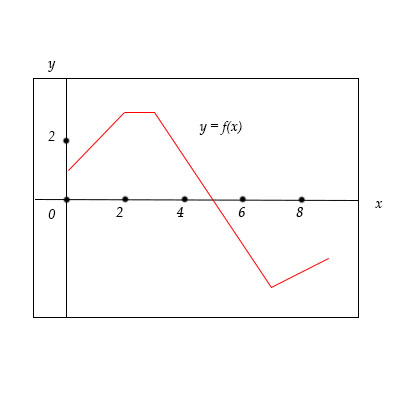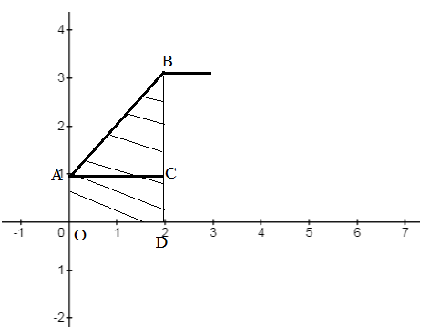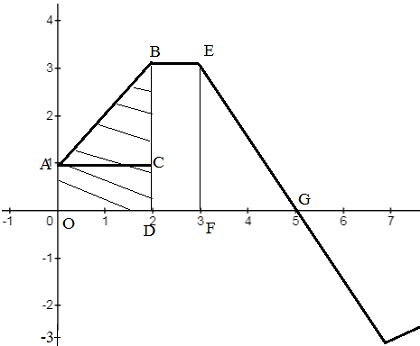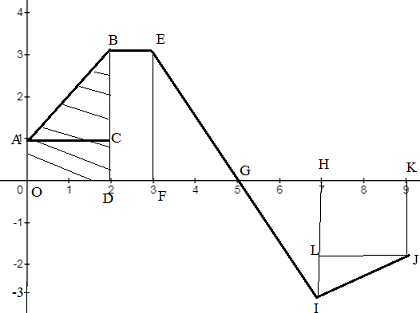# The graph of f is shown below. Evaluate each integral by interpreting in therms of areas. a....

## Question:

The graph of {eq}f {/eq} is shown below. Evaluate each integral by interpreting in therms of areas.

a. {eq}\int_0^2 f(x) dx {/eq}

b. {eq}\int_0^5 f(x) dx {/eq}

c. {eq}\int _0^7 f(x) dx {/eq}

d. {eq}\int_0^9 f(x) dx {/eq}## Sum of Areas:

According to the bounded regions or required sum of these regions, we'll compute the exact value of the given different definite integrals. For that, we need to use the general formulas of the area of the right-angle triangle and the rectangle shown below:

{eq}\displaystyle \textrm{Area of triangle}=\frac{1}{2}\times \textrm{perpendicular}\times \textrm{base}\\ \displaystyle \textrm{Area of rectangle}=\displaystyle \textrm{length}\times \textrm{width} {/eq}

a.

The given integral expression is:

{eq}\int_0^2 f(x) dx=? {/eq}

According to the boundaries of the above integral expression and the given graph of the function, the bounded regions in the graph is:According to the above figure, we'll rewrite the given integral expression as:

{eq}\displaystyle \int_0^2 f(x) dx=\textrm{Area of ABC}+\textrm{Area of OACD}........(1) {/eq}

The value of the area of the triangle ABC is:

{eq}\begin{align*} \displaystyle \textrm{Area of ABC}&=\frac{1}{2}\times AC\times BC\\ &=\frac{1}{2}\times 2\times2\\ &=2 \end{align*} {/eq}

The value of the area of the rectangle OACD is:

{eq}\begin{align*} \displaystyle \textrm{Area of OACD}&=OA\times OD\\ &=1\times 2\\ &=2 \end{align*} {/eq}

Plugging above values in equation (1) and simplifying it, we get:

{eq}\begin{align*} \displaystyle \int_0^2 f(x) dx&=2+2\\ \displaystyle \int_0^2 f(x) dx&=\boxed4\\ \end{align*} {/eq}

b.

The given integral expression is:

{eq}\displaystyle \int_0^5 f(x) dx=? {/eq}

According to the boundaries of the above integral expression and the given graph of the function, the bounded regions in the graph is:According to the above figure, we'll rewrite the given integral expression as:

{eq}\begin{align*} \displaystyle \int_0^5 f(x)\ dx&=\textrm{Area of ABC}+\textrm{Area of OACD}+\textrm{Area of BDEF}+\textrm{Area of EFG}\\ &= \int_0^2 f(x) dx+\textrm{Area of BDEF}+\textrm{Area of EFG}\\ &=4+\textrm{Area of BDEF}+\textrm{Area of EFG}.........(2)\\ \end{align*} {/eq}

The value of the area of the triangle BDEF is:

{eq}\begin{align*} \displaystyle \textrm{Area of BDEF}&=BD\times DF\\ \displaystyle \textrm{Area of BDEF}&=3\times 1\\ &=3\\ \end{align*} {/eq}

The value of the area of the triangle EFG is:

{eq}\begin{align*} \displaystyle \textrm{Area of EFG}&=\frac{1}{2}\times EF\times FG\\ \displaystyle \textrm{Area of EFG}&=\frac{1}{2}\times 3\times 2\\ &=3\\ \end{align*} {/eq}

Plugging above values in equation (2) and simplifying it, we get:

{eq}\begin{align*} \displaystyle \int_0^5 f(x)\ dx&=4+\textrm{Area of BDEF}+\textrm{Area of EFG}\\ &=4+3+3\\ &=\boxed{10}\\ \end{align*} {/eq}

c.

The given integral expression is:

{eq}\int _0^7 f(x) dx=? {/eq}

According to the boundaries of the above integral expression and the given graph of the function, the bounded regions in the graph is:According to the above figure, we'll rewrite the given integral expression as:

{eq}\begin{align*} \displaystyle \int_0^7 f(x)\ dx&=\textrm{Area of ABC}+\textrm{Area of OACD}+\textrm{Area of BDEF}+\textrm{Area of EFG}-\textrm{Area of GHI}\\ &= \int_0^2 f(x) dx+\textrm{Area of BDEF}+\textrm{Area of EFG}-\textrm{Area of GHI}\\ &=4+\textrm{Area of BDEF}+\textrm{Area of EFG}-\textrm{Area of GHI}\\ &=\int_0^5 f(x)\ dx-\textrm{Area of GHI}\\ &=10-\textrm{Area of GHI}...................(3)\\ \end{align*} {/eq}

We are subtracting the area of triangle GHI as it is below the x-asix.

The value of the area of the triangle GHI is:

{eq}\begin{align*} \displaystyle \textrm{Area of GHI}&=\frac{1}{2}\times GH\times HI\\ \displaystyle \textrm{Area of GHI}&=\frac{1}{2}\times 2\times 3\\ &=3\\ \end{align*} {/eq}

Therefore,

{eq}\begin{align*} \displaystyle \int_0^7 f(x)\ dx&=10-\textrm{Area of GHI}\\ &=10-3\\ &=\boxed{7} \end{align*} {/eq}

d.

The given integral expression is:

{eq}\int_0^9 f(x) dx=? {/eq}

According to the figure in part (c), we'll rewrite the above integral expression as:

{eq}\begin{align*} \displaystyle \int_0^9 f(x)\ dx&=\displaystyle \int_0^7 f(x)\ dx-(\textrm{area of HKJL}+\textrm{area of LJI})\\ &=\displaystyle 7-\textrm{area of HKJL}-\textrm{area of LJI}............(4) \end{align*} {/eq}

The value of the area of the triangle LJI is:

{eq}\begin{align*} \displaystyle \textrm{Area of LJI}&=\frac{1}{2}\times LJ\times LI\\ \displaystyle \textrm{Area of LJI}&=\frac{1}{2}\times 2\times 1\\ &=1\\ \end{align*} {/eq}

The value of the area of the Square HKJL is:

{eq}\begin{align*} \displaystyle \textrm{Area of HKJL}&= HK\times HL\\ \displaystyle \textrm{Area of HKJL}&=2\times 2\\ &=4\\ \end{align*} {/eq}

By the above values and equation (4), we have:

{eq}\begin{align*} \displaystyle \int_0^9 f(x)\ dx&=\displaystyle 7-\textrm{area of HKJL}-\textrm{area of LJI}\\ &=\displaystyle 7-4-1\\ &=\displaystyle 7-5\\ &=\displaystyle \boxed{2}\\ \end{align*} {/eq}